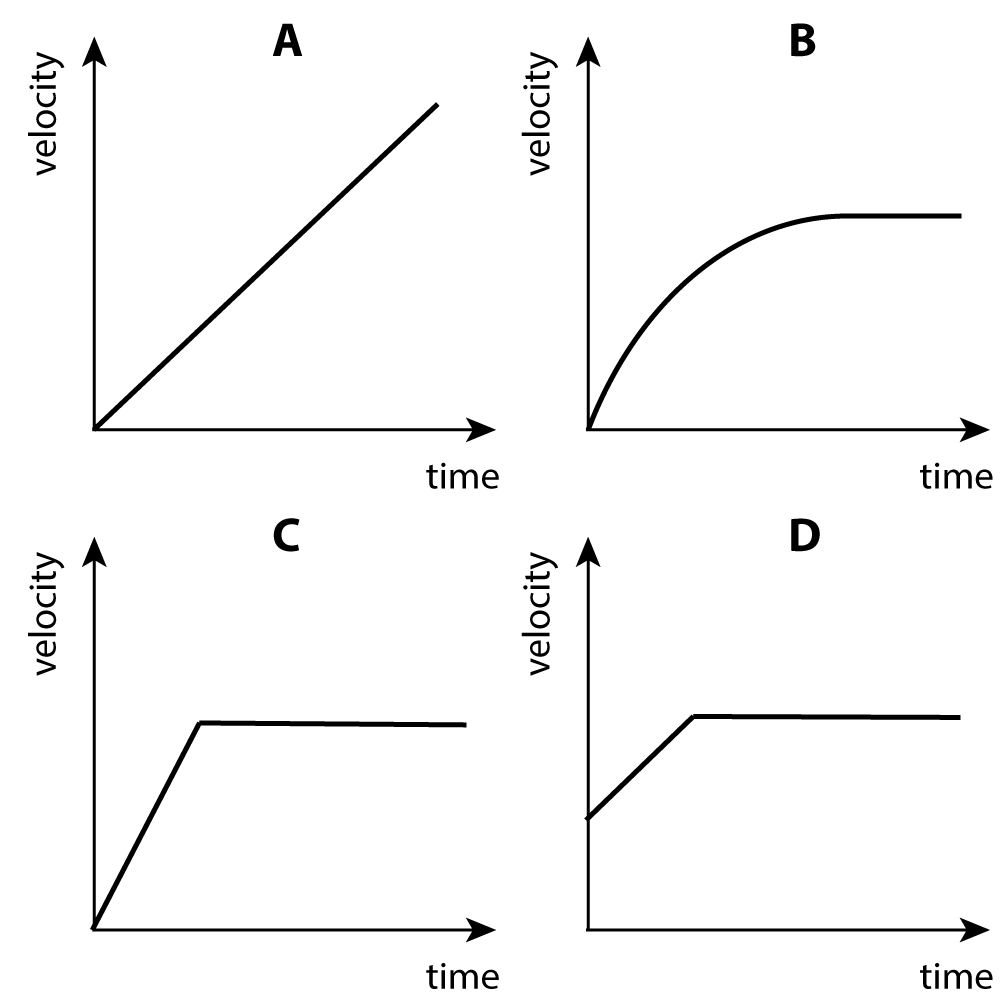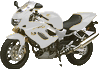Choose the correct units for the following measurements :
1. Mass
2. Weight
3. Velocity
4. Friction

5-8: The following questions are about a parachute jump.

A skydiver jumps out of a plane. Gravity pulls the person downwards. Air resistance pushes upwards. Later on they open the parachute.

They are shown here at the bottom of the jump.

Choose the sentences that best describe what happens:5. Just after jumping out and before the parachute has been opened...

• A). The air resistance decreases
• B). The force of gravity increases as the person accelerates
• C). The upward force increases
• D). The 2 forces are always balanced

6. After a while the jumper reaches terminal velocity. This happens when

• A). the air resistance and the force of gravity are balanced
• B). the force of gravity increases more than the air resistance
• C). air resistance is the largest force
• D). the person is accelerating at a constant rate.

7. Which of these graphs shows the motion up to this point?8. The person now opens his parachute. What happens now?

• A). the air resistance and the force of gravity are balanced
• B). the force of gravity decreases
• C). the resultant force is now upwards
• D). the person moves upwards as air resistance increases

9+10: A motorbike moves along a road at 25m/s.

Calculate ..9. The distance moved in 50s.

• A). 2 m
• B). 0.5 m
• C). 125 m
• D). 1.25 km

10. The time taken to travel 2 kilometres.

• A). 4 s
• B). 80 s
• C). 0.08 s
• D). 40 s

11. A train accelerates from 10 m/s to 50 m/s in 20 seconds. The acceleration is:

• A). 2 m/s2
• B). 2.5 m/s2
• C). 800 m/s2
• D). 1000 m/s2

In a formula 1 car race the acceleration of the first few cars was measured from the start grid. What are the missing values?

 Car Starting velocity (m/s) Final velocity (m/s) Time taken (s) acceleration (m/s2) 12 Ferrari 5 30 ? 0 5 6 25 130 750 5 13 McClaren 0 ? 72 36 2 0.5 12 6